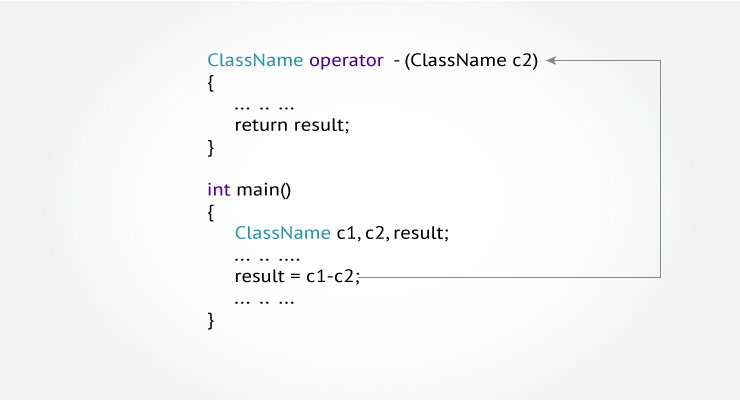In C++, it's possible to change the way operator works (for user-defined types). In this article, you will learn to implement operator overloading feature.The meaning of an operator is always same for variable of basic types like: int, float, double etc. For example: To add two integers, + operator is used.

However, for user-defined types (like: objects), you can redefine the way operator works. For example:

If there are two objects of a class that contains string as its data members. You can redefine the meaning of + operator and use it to concatenate those strings.

This feature in C++ programming that allows programmer to redefine the meaning of an operator (when they operate on class objects) is known as operator overloading.

You can write any C++ program without the knowledge of operator overloading. However, operator operating are profoundly used by programmers to make program intuitive. For example,

You can replace the code like:

calculation = add(multiply(a, b),divide(a, b));

to

calculation = (a*b)+(a/b);

How to overload operators in C++ programming?

To overload an operator, a special operator function is defined inside the class as:

class className
{
... .. ...
public
returnType operator symbol (arguments)
{
... .. ...
}
... .. ...
};
• Here, returnType is the return type of the function.
• The returnType of the function is followed by operator keyword.
• Symbol is the operator symbol you want to overload. Like: +, <, -, ++
• You can pass arguments to the operator function in similar way as functions.

#include <iostream>
using namespace std;

class Test
{
private:
int count;

public:
Test(): count(5){}

void operator ++()
{
count = count+1;
}
void Display() { cout<<"Count: "<<count; }
};

int main()
{
Test t;
// this calls "function void operator ++()" function
++t;
t.Display();
return 0;
}

Output

Count: 6

This function is called when ++ operator operates on the object of Test class (object t in this case).

In the program,void operator ++ () operator function is defined (inside Test class).

This function increments the value of count by 1 for t object.

Things to remember

1. Operator overloading allows you to redefine the way operator works for user-defined types only (objects, structures). It cannot be used for built-in types (int, float, char etc.).
2. Two operators = and & are already overloaded by default in C++. For example: To copy objects of same class, you can directly use = operator. You do not need to create an operator function.
3. Operator overloading cannot change the precedence and associatively of operators. However, if you want to change the order of evaluation, parenthesis should be used.
4. There are 4 operators that cannot be overloaded in C++. They are :: (scope resolution), . (member selection), .* (member selection through pointer to function) and ?: (ternary operator).

Following best practices while using operator overloading

Operator overloading allows you to define the way operator works (the way you want).

In the above example, ++ operator operates on object to increase the value of data member count by 1.

void operator ++()
{
count = count+1;
}

However, if you use the following code. It decreases the value of count by 100 when ++ operator is used.

void operator ++()
{
count = count-100;
}

This may be technically correct. But, this code is confusing and, difficult to understand and debug.

It's your job as a programmer to use operator overloading properly and in consistent way.

In the above example, the value of count increases by 1 when ++ operator is used. However, this program is incomplete in sense that you cannot use code like:

t1 = ++t

It is because the return type of the operator function is void.

Visit these pages to learn more on: